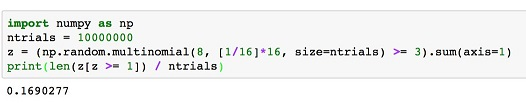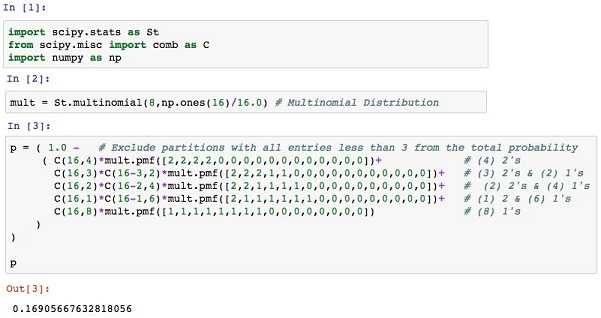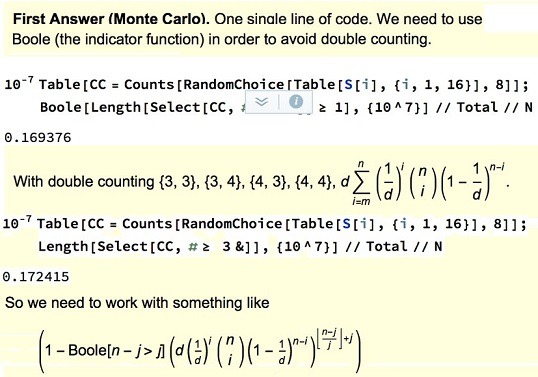# Practical Inevitability of Clustering

### Problem### N. N. Taleb's General Solution

We start with the general. The compositions of a positive integer $n$ into $d$ ordered parts treating $0$ as a significant addend (e.g. $0+3$ is different from $3+0)$ is given by the vector $C_{p,k}$. The number of compositions will be:

$\displaystyle \frac{(d+n-1)!}{(d-1)! n!}$

More specifically

$\displaystyle \mathcal{M}_{(n,\overset{\rightharpoonup }{p})}(\overset{\rightharpoonup }{\chi _i})= n! \prod _{j=1}^d \frac{p_j^{(\overset{\rightharpoonup }{\chi _i})_j}}{(\overset{\rightharpoonup }{\chi _i})_j!}$

We finally have

$\displaystyle \mathbb{P}(M\geq m)=\sum _{i=1}^{\frac{(d+n-1)!}{(d-1)! n!}} \mathbb{1}_{\max \overset{\rightharpoonup }{\chi _i}\geq m}\mathcal{M}_{\left(n,\overset{\rightharpoonup }{p}\right)}\left(\overset{\rightharpoonup }{\chi _i}\right)$

On our example, using Mathematica code with the package Combinatorica:

\displaystyle \chi =\text{Compositions}(8,16);\\ \begin{align} \sum _{i=1}^{\text{Length}[\chi ]} \text{Boole}[\max (\chi [[i]])&\geq 3] \text{PDF}[\text{MultinomialDistribution}[8,p],\chi [[i]]]\\ &\approx 0.169376. \end{align}

### Steven Morse's Illustration And Code### Amit Atagi's Code### N. N. Taleb's Combinatorial Simulation### Long Huynh Huu's Enumerative Combinatorics

We compute the probability $p'$ that every square has $\lt m$ darts, then find $p = 1-p'.$ Let's say we're given a vector $(k_1,\ldots,k_d)$ saying that $k_i$ darts hit the $i^{th}$ square. Then we can compute its class $(n_0,\ldots ,n_{m-1})$ where $n_i = |\{j\mid k_j = i\}|$ counts the number of squares with $i$ darts. The probability that $k_i$ darts hit the $i^{th}$ square then is

\begin{align} \binom{n}{k_1;\ldots ;k_d}n^{-d} = \frac{n!}{0!^{n_0}\cdot \ldots \cdot (m-1)!^{n_{m-1}}}n^{-d}, \end{align}

so it depends only on the class. The set of all classes is

$\displaystyle \mathfrak{C}(d,n,m) := \{ (n_0,\ldots ,n_{m-1}) \in \mathbb{N}_0^m \mid \sum_i n_i = d, \sum_i in_i = n \}$

For instance

$\mathfrak{C}(16,8,3) = \{(8,8,0),(9,6,1),(10,4,2),(11,2,3),(12,0,4)\}$

For each class $(n_0,\ldots ,n_{m-1})$ there are $\binom{d}{n_0;\ldots ;n_{m-1}}$ distributions of darts $(k_1,\ldots ,k_d).$ We get

$\displaystyle p' = \sum_{(n_0,\ldots ,n_{m-1})\in\mathfrak{C}(d,n,m)} \binom{d}{n_0;\ldots ;n_{m-1}} \frac{n!}\prod_{i=0}^{m-1} (i!)^{n_i}}d^{-n}$

        import Data.Ratio

classes d 0 1 = [[d]]
classes d n 1 = []
classes d n m =
let r = div n (m-1)
in  concatMap (\ k -> map (++[k])$classes (d-k) (n - k*(m-1)) (m-1)) [0..r] cluster_probability d n m = let f ns = (factorial d * factorial n) % ((product (map factorial ns)) *(product (zipWith (^) (map factorial [0..m-1]) ns)) *(d^n)) in 1 - sum (map f (classes d n m))  ### Probabilistic Pigeonhole Principle A probabilistic generalization of the pigeonhole principle states that if$n$pigeons are randomly put into$m$pigeonholes with uniform probability$\displaystyle \frac{1}{m},$then at least one pigeonhole will hold more than one pigeon with probability$\displaystyle 1-\frac{(m)_n}{m^n},$where$(m)_n$is the falling factorial$m(m-1)\cdot(m-n+1).$For$n=0$and for$n=1$(and$m\gt 0),$that probability is zero; in other words, if there is just one pigeon, there cannot be a conflict. For$n\gt m$(more pigeons than pigeonholes) it is one, in which case it coincides with the ordinary pigeonhole principle. But even if the number of pigeons does not exceed the number of pigeonholes$(n\le m),$due to the random nature of the assignment of pigeons to pigeonholes there is often a substational chance that clashes will occur. For example, if$2$pigeons are randomly assigned to$4$pigeonholes, there is a$25%$chance that at least one pigeonhole will hold more than one pigeon; for$n=5, m=10$the probability is$69.76$and for$n=10,m=20$it is$93.45%.$### Quote Here's from pp 169-170 of Fooled by Randomness by N. N. Taleb: I would further illustrate the point with study of the phenomenon well-known as cancer-clusters. Consider a square with 16 random darts hitting it with equal probability of being at any place in the square. If we divide the square into 16 smaller squares, it is expected that each smaller square will contain one dart on average - but only on average. There is a very small probability of having 16 darts in 16 different squares. The average grid will have more than one dart in a few squares and no dart at all in many squares. It will be an exceptionally rare incident that no (cancer) cluster would show on the grid. Now, transpose our grid with the darts on it to overlay a map of any region. Some newspapers will declare that one of the areas (the one with more than the average of the darts) harbors radiation that causes cancer, prompting lawyers to start soliciting the patients. ### Acknowledgment The question has been posted on twitter by N. N. Taleb where I collected some of the responses in the ensuing discussion. In addition, Mike Lawler analyzed the problem in his blog. The reason for raising the question is well explained in the above quote from Taleb's book Fooled by Randomness. During the discussion Long Huynh Huu had referred to the online resource Enumerative Combinatorics by Anna de Mier. Marcos Carreira brought into the discussion the generalized (probabilistic) pigeonhole principle. One of the tweets linked to a math.stackexchange discussion on the probability of three people sharing a birthday in a room of$30.\$